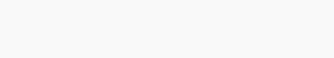# Ex 9.2 Class 8 Maths Solutions from NCERT - Learn Hool

 Board CBSE Textbook NCERT Class Class 8 Subject Maths Chapter Chapter 9 Chapter Name Algebraic Expressions and Identities Exercise Ex 9.2 Number of Questions Solved 5 Category NCERT Solutions

## NCERT Solutions for Class 8 Maths Chapter 9 Algebraic Expressions and Identities Ex 9.2

Exercise 9.2 Class 8 Question 1.
Find the product of the following pairs of monomials:
(i) 4, 7p
(ii) – 4p, 7p
(iii) – 4p, 7pq
(iv) $$4{ p }^{ 3, },\quad -3p$$
(v) 4p, 0.
Solution.Exercise 9.2 Class 8 Question 2.
Find the areas of rectangles with the following pairs of mononials as their lengths and breadths respectively:
(i) (p, q);
(ii) (10m, 5n);
(iii) $$(20{ x }^{ 2 },\quad 5{ y }^{ 2 })$$;
(iv) $$(4x,\quad 3{ x }^{ 2 })$$;
(v) (3mn, 4np).
Solution.
(i) (p, q)
Length = p
∴ Area of the rectangle
= pxq
= pq

(ii) (10m, 5n)
Length = 10 m
∴ Area of the rectangle
= (10m) x (5n)
= (10 x 5) x (m x n)
= 50 x (mn)
= 50 mn

(iii) $$(20{ x }^{ 2 },\quad 5{ y }^{ 2 })$$
Length = (20{ x }^{ 2 })
Breadth = (5{ y }^{ 2 })
∴ Area of the rectangle
= $$(20{ x }^{ 2 })$$ x $$(5{ y }^{ 2 })$$
= (20 x 5) x $$({ x }^{ 2 }\times { y }^{ 2 })$$
= 100 x $$({ x }^{ 2 }{ y }^{ 2 })$$
= 100$${ x }^{ 2 }{ y }^{ 2 }$$

(iv) (4x, 3xP)
Length = 4.x
Breadth = (3{ x }^{ 2 })
∴ Area of the rectangle
(4x) x $$(3{ x }^{ 2 })$$
= (4 x 3) x $$(x\times { x }^{ 2 })$$
= 12 x $${ x }^{ 3 }$$
= 12×3

(v) (3mn, 4np)
Length = 3 mn
∴ Area of the rectangle
= (3mn) x (4np)
= (3 x 4) x (mn) x (np)
= 12 x m x (n x n) x p
= 12$$m{ n }^{ 2 }p$$

Exercise 9.2 Class 8 Question 3.
Complete the table of products.Solution.Exercise 9.2 Class 8 Question 4.
Obtain the volume of rectangular boxes with the following length, breadth and height respectively:
(i) $$5a,\quad 3{ a }^{ 2 },\quad 7{ a }^{ 4 }$$
(ii) 2p, 4q, 8r
(iii) $$xy,\quad 2{ x }^{ 2 }y,\quad 2x{ y }^{ 2 }$$
(iv) a, 2b, 3c
Solution.
(i) $$5a,\quad 3{ a }^{ 2 },\quad 7{ a }^{ 4 }$$(ii) 2p, 4q, 8r(iii) $$xy,\quad 2{ x }^{ 2 }y,\quad 2x{ y }^{ 2 }$$(iv) a, 2b, 3cExercise 9.2 Class 8 Question 5.
Obtain the product of
(i) xy, yz, zx
(ii) $$a,\quad -{ a }^{ 2 },\quad { a }^{ 3 }$$
(iii) $$2,\quad 4y,\quad 8{ y }^{ 2 },\quad 16{ y }^{ 3 }$$
(iv) a, 2b, 3c, 6abc
(v) m, – mn, mnp.
Solution.
(i) xy, yz, zx
Required product
= (xy) x (yz) x (zx)(ii) $$a,\quad -{ a }^{ 2 },\quad { a }^{ 3 }$$(iii) $$2,\quad 4y,\quad 8{ y }^{ 2 },\quad 16{ y }^{ 3 }$$(iv) a, 2b, 3c, 6abc(v) m, – mn, mnp.We hope the NCERT Solutions for Class 8 Maths Chapter 9 Algebraic Expressions and Identities Ex 9.2 help you. If you have any queries regarding NCERT Solutions for Class 8 Maths Chapter 9 Algebraic Expressions and Identities Ex 9.2, drop a comment below and we will get back to you at the earliest.

@ Designed By : Vikas Copyright 2023 @ LearnHool.In